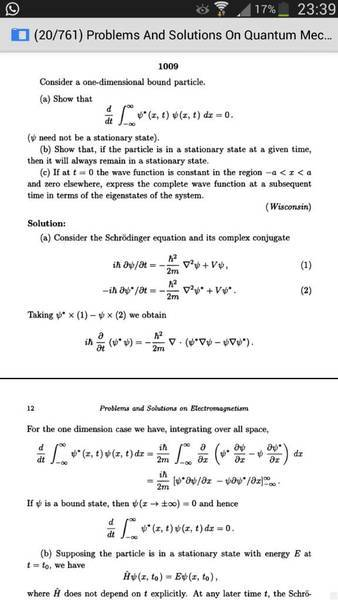# Concepts about infinite potential well

• VHAHAHA

#### VHAHAHAAs we know, the 1d infinite potential well has a stationary state. The function that depends on x onky is a sin function.
However, I don't understand the concept in this question. I have the answer of this question and this is not a homework. I am not asking for the answer so please don't put this post to the homework section.
I don't understand the part 2 of this question because the potential well should be in stationary state. Why i need to prove it in part 2?
Also, in part 3, i don't understand why there is a constant function. The fuction should be a product of sin function and exponential function. How come there is a constant function?

The authors are asking you to expand a constant function in a Fourier sine series. It's a constant in the sense that it does not depend on the position for a fixed time t=0. For subsequent times, it won't be a constant anymore.

dauto said:
The authors are asking you to expand a constant function in a Fourier sine series. It's a constant in the sense that it does not depend on the position for a fixed time t=0. For subsequent times, it won't be a constant anymore.

what is a Fourier seriesVHAHAHA said:
what is a Fourier seriesA sum of sines and/or cosines whose frequencies are integer multiples of a fundamental frequency (if we're talking about a function of time) or whose wavenumbers are integer multiples of a fundamental. See for example

http://en.wikipedia.org/wiki/Fourier_series

what is the relation between these two ?:(

See, Simply put , stationary states of 1-d box are those in which the probability density (and also expectation values) are independent of time. do this : construct the time dependent wavefunction by stacking exponential time dependence alongside the sines. check out the probablity density. in this case multiplication of complex exponentail (in time) and its conjugate causes time dependence part to fizzle out, leaving only sine squared. ditto with avg. value of any operator.
QED

VHAHAHA said:
what is the relation between these two ?:(

I'm guessing that by "these two" you mean Fourier series and the infinite square well.

The stationary states of the infinite square well (0 < x < a) are
$$\Psi_n(x,t) = \sqrt{\frac{2}{a}}\sin \left( \frac{n \pi x}{a} \right) e^{-i E_n t / \hbar}$$

Any state of the infinite square well can be written as a linear combination of these:
$$\Psi(x,t) = \displaystyle\sum_{n=1}^\infty {c_n \Psi_n(x,t)}$$

At t = 0:
$$\Psi(x,0) = \displaystyle\sum_{n=1}^\infty {c_n \sqrt{\frac{2}{a}}\sin \left( \frac{n \pi x}{a} \right)}$$

which is basically a Fourier series. For any function defined in the range 0 < x < a, you can find the coefficients ##c_n## which make this true. Put these coefficients into the linear combination for ##\Psi(x,t)## and it tells you how the wave function evolves in time from ##\Psi(x,0)##. Any decent QM textbook has the details.